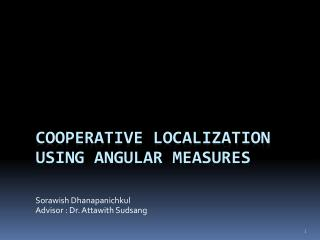# Cooperative Localization using angular measures - PowerPoint PPT PresentationDownload PresentationCooperative Localization using angular measures

Cooperative Localization using angular measuresDownload Presentation## Cooperative Localization using angular measures

- - - - - - - - - - - - - - - - - - - - - - - - - - - E N D - - - - - - - - - - - - - - - - - - - - - - - - - - -
##### Presentation Transcript

1. Sorawish Dhanapanichkul Advisor : Dr. AttawithSudsang Cooperative Localization using angular measures

2. Our problem • Localization • Multi-robot localization

3. Our problem

4. Our problem

5. Our problem • Output • A Positional pattern • Input • Angular measurements A11,…,A14 A21,…,A24 (X1,Y1) (X2,Y2) Cooperative Localization Algorithm A31,…,A34 A41,…,A44 (X3,Y3) A51,…,A54 (X5,Y5) (X4,Y4)

6. Our problem Line Of Sight(LOS)

7. Introduction to problem • Unknown correspondence between measurements and robots’ ID

8. Introduction to problem • Matching Problem • Between measurements and robots’ name Naïve O(NN)

9. Proposed algorithms • Geometric based algorithm • Based on triangulation • Sensitive to noise • Convex optimization based algorithm • Transform the problem to convex optimization problem • More flexibility

10. Scope • 2D planar space • Fully visible • Include Uncertainty (measurement’s noise)

11. Geometric based algorithm • Use property of convex hull • Reduce matching complexity • Based on triangulation

12. Triangulation • Example • 3 robots • 2 known coordinatesand 1 LOS 3 1 2

13. Triangulation : Ghost node • > 3 robots (ex. 4 robots) 4 3 1 2

14. Ghost node elimination • 1 more known coordinate + 2 LOSs 4 3 coordinates 3 LOSs 3 1 2 5

15. Geometric based algorithm • Compute the position of 3 robots • Triangulation using angular measures of 3 robots with known position

16. Our matching algorithm • Convexity of LOS Graph 4 Boundary lines 3 1 2 5 Boundary points

17. Define set of boundary point • A boundary point • have 2 measurements which all the others reside between these two measurements • These 2 measurements are called leftmost(LM) and rightmost(RM) • RM  LM <= 180 degrees LM1 RM2 4 RM4 LM4 1 2 LM5 5 RM5 LM2 RM1

18. Find the boundary points which connected with that reference • Choose reference point • From set of boundary points • By using LM and RM • Set the distance between one of them to be 1 1 2 RM1 1 LM2 5 LM5 RM5 (0,0)

19. Find last LOS • Try to find the last LOS • To find the last coordinate 1 2 1 5 (0,0)

20. Find last LOS • These 3 robots are forming a triangle • Assuming that there are S robots inside this triangle Convex!!! S S+1 1 2 1 5 (0,0)

21. Find last LOS • Example: S = 3 • After sorting, compare 1st measurement of robot 1 and 2 3 1 2 1 5 (0,0)

22. Find last LOS • Example: S = 3 • After sorting, compare 2nd measurement of robot 1 and 2 3 1 2 1 5 (0,0)

23. Find last LOS • Example: S = 3 • After sorting, compare 3rd measurement of robot 1 and 2 3 1 2 1 5 (0,0)

24. Find last LOS • Example: S = 3 • After sorting, compare 4th measurement of robot 1 and 2 3 3+1 1 2 1 5 (0,0)

25. Algorithm summary • Use our matching algorithm to find 3 LOS • Calculate all intersection from 2 robots • Use 3rd robot’s measurements to eliminate ghost node Time complexity : O(N2) + O(N2) + O(N2 lg(N)) 1 2 5

26. Measurement noise • Due to our comparing method (opposite direction) • Change comparing method

27. Ghost returns • Special case Ex. 6 robots Ghost node

28. Experimental result Wrong rate Number of robot

29. Convex optimization based algorithm

30. Convex optimization based algorithm • Propose iterative method :: try to minimize error • Reduce problem to convex optimization problem

31. Iterative method - flow • Random the answer • Update new answer by step vector • Meet termination condition Yes No End

32. Iterative method - Example 0th step -Random Actual Answer B A C C A B

33. Iterative method - Example 1st step Actual Answer B A C C A B

34. Iterative method - Example 2nd step Actual Answer B A C C B A

35. Iterative method - Example 3rd step Actual Answer B A B C C A

36. Iterative method - Example Termination condition Actual Answer B B C C A A

37. Step vector • Difference between “Sum vector” of actual and answer • Ex. Sum vector A A 0th step Actual Answer B A Step vector A C C A B

38. Example • C# Answer Actual

39. Example • C# Answer Actual

40. Error Actual errorAB • Total error = Σerrorij • Mean angular error = total error / no. of input B B Result A A

41. Experimental result Mean angular error (Radian) Number of robot

42. Mathematical explanation • Compare iterative method with gradient descent • Proof of correctness

43. Update eqn <-> Gradient descent Optimization problem !!! • Update equation • Gradient descent

44. Integration of gradient • Gradient of function • After integration

45. Convex function • Property • One lowest value • Locally optimal point = Globally optimal point Convex!!

46. Proof of correctness • Rewrite the equation (no error) • Lowest value • All robots in the result are at the same place • vector from robot ith to jth of the result = vector from robot ith to jth of the real robot

47. Experimental result 3.437 degrees Mean angular error (Radian) Number of robot

48. Experimental result Number of iteration step Number of robot

49. Algorithm summary • Reduce problem  Convex optimization • Tolerate to measurement noise

50. Conclusion